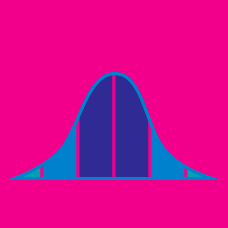Probability

# Standard Deviation

If the standard deviation of the following probability distribution: $\begin{array}{c}&P(X = 0) = 0.2, &P(X = 1) = 0.4, &P( X = 2) = 0.4\end{array}$ is $A,$ what is the value of $A^2?$

If the standard deviation of the following probability distribution: $\begin{array}{c}&P(X = 0) = 0.1, &P(X = 1) = 0.3, &P( X = 2) = 0.6 \end{array}$ can be expressed as $\frac{1}{10} \sqrt{A},$ what is $A?$The figure above represents the graphs of the probability density functions of three different probability distributions that have the same mean $m.$ Which of the following correctly represents them in order of increasing standard deviation?

Note: Assume that the areas under the curves are all equal to 1.

If the standard deviation of the numbers $19, 21, 22$ and $23$ is a constant $m$, then the standard deviation of the numbers $21, 23, 24$ and $25$ is $\text{\_\_\_\_\_\_\_\_\_}.$

What is the standard deviation of the following probability distribution: $\begin{array}{c}&P(X = 4) = \frac{1}{2}, &P( X = 14) = \frac{1}{2} ? \end{array}$

×